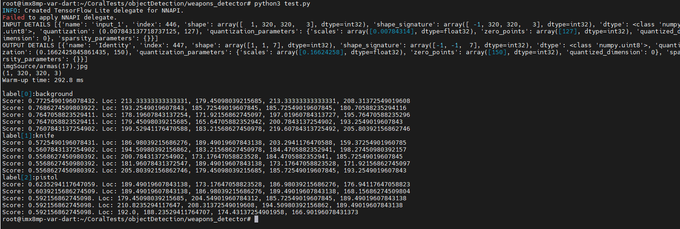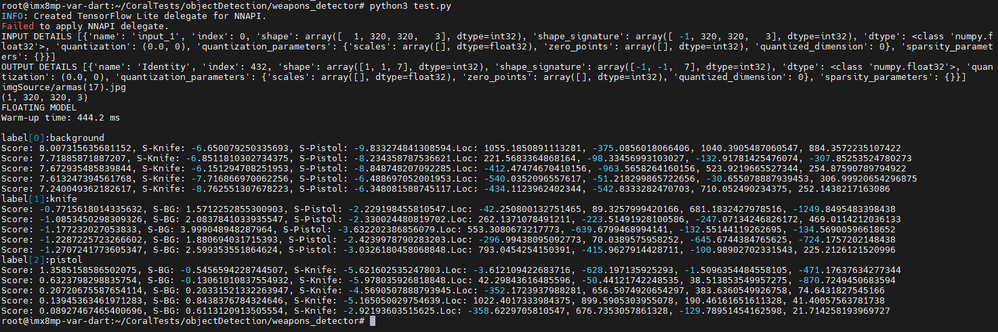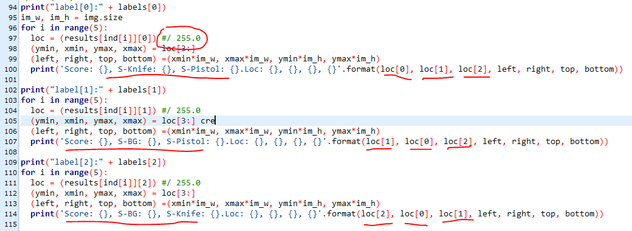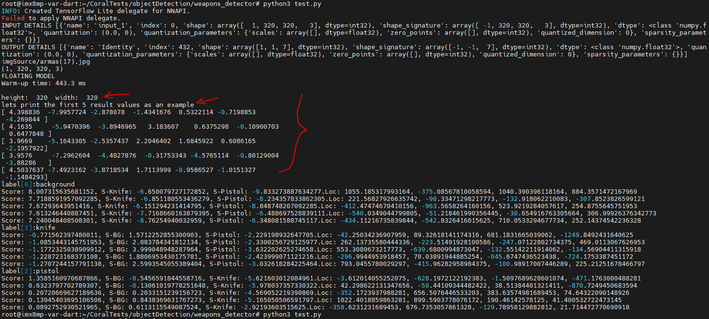# How to interpret the output from a mobilenet_V3 correctly?

cancel
Showing results for
Show  only  | Search instead for
Did you mean:

## How to interpret the output from a mobilenet_V3 correctly?

5,977 ViewsContributor II

Hello,

I have successfully trained a ssd_mobilenet_V3 with the scale set to small and the Activation set to relu6.

I exported the model to a .h5 format (other options are also possible). When I execute model.predict(...)
I recieve a matrix with the dimension [1,2034,12]. The first dimension is the batch size, the second one is the amount of bounding boxes detected. The last dimension is the result for the classes and the combination for the bounding box. I have 8 classes (7 + Background) , so I assume that the first eight entries are the classes, followed by some kind of definition for the bounding box.

I am able to sort out the background and only have the hits for the classes available, however the bounding box information looks like this:

[ 0.3028022 1.5857686 -1.8396118 -2.9573815]
[-0.41526774 1.8189278 -1.2391882 -2.7607949 ]
[ 0.09888815 -1.2759354 -2.472039 -4.031958 ]
[ 1.2891976 0.5669114 -3.1997669 -5.219625 ]
[-1.9375236 0.81477445 -1.27267 -3.0619001 ]
[-0.5534759 -0.83221847 -2.0240316 -3.2735825 ]
[-1.7881895 1.1753309 -2.5431674 -3.924986 ]
[ 1.7990638 -1.0902078 -3.4435217 -4.631519 ]
[-1.8851086 0.63339823 -2.3908594 -3.011328 ]

My Input Image has the size of [320,320,3] - how do I need to transform these Outputs in order to create the correct bounding box for each line?

In the demo.c file from the eIQ DeepViewRT GStreamer Detection Demo I was able to find the functions  nn_ssd_decode_nms_standard_bbx and nn_ssd_decode_nms_variance_bbx . I am assuming that they are doing what I would like to do in Python, offline from the Board.

Can please someone from NXP / AU-Zone share what these function does in detail? This is a crucial part in development and needs to be adressed in order to use eIQ Portal in future.

Regards,

Kevin

Labels (2)

• ### i.MX RT

12 Replies
3,564 ViewsContributor I

Did anyone succeed facing this issue?

5,490 ViewsContributor I

Hi @Ramson,

In principle what you said may sound good to me. I trained an object detector model with the eIQ portal based on the ssd_mobilenet_V3 and my own data set. My model considers two possible classes as output (plus the background?). The output data provides consequently a [1,1,7] tensor:

[1,1,4]-> object location
[1,1,5]-> background score as you suggest or class type as suggested here by MarcinChelminsk (‎09-06-2021 06:45 AM)
[1,1,6-7]-> corresponding to scores of my two main classesHowever, after invoking the inference, I am not able to interpret the output at all. My model, same as yours, results on  [1,2034,7] 2034 possible objects found.

...
interpreter = tflite.Interpreter('detection-balanced-mcu-2021-11-15T14-20-27.767Z_in-uint8_out-uint8_channel_mlir_ptq.tflite')
interpreter.allocate_tensors()

input_details = interpreter.get_input_details()
print("INPUT DETAILS", input_details)
output_details = interpreter.get_output_details()
print("OUTPUT DETAILS", output_details)

INPUT DETAILS [{'name': 'input_1', 'index': 446, 'shape': array([ 1, 320, 320, 3], dtype=int32), 'shape_signature': array([ -1, 320, 320, 3], dtype=int32), 'dtype': <class 'numpy.uint8'>, 'quantization': (0.007843137718737125, 127), 'quantization_parameters': {'scales': array([0.00784314], dtype=float32), 'zero_points': array(, dtype=int32), 'quantized_dimension': 0}, 'sparsity_parameters': {}}]
OUTPUT DETAILS [{'name': 'Identity', 'index': 447, 'shape': array([1, 1, 7], dtype=int32), 'shape_signature': array([-1, -1, 7], dtype=int32), 'dtype': <class 'numpy.uint8'>, 'quantization': (0.1662425845861435, 150), 'quantization_parameters': {'scales': array([0.16624258], dtype=float32), 'zero_points': array(, dtype=int32), 'quantized_dimension': 0}, 'sparsity_parameters': {}}]

# NxHxWxC, H:1, W:2
height = input_details['shape']
width = input_details['shape']
img = Image.open('imgSource/armas(1).jpg').resize((height,width ))

input_data = np.expand_dims(img, axis=0)
interpreter.invoke()

output_details = interpreter.get_output_details()
print("OUTPUT DETAILS", output_details)

OUTPUT DETAILS [{'name': 'Identity', 'index': 447, 'shape': array([ 1, 2034, 7], dtype=int32), 'shape_signature': array([-1, -1, 7], dtype=int32), 'dtype': <class 'numpy.uint8'>, 'quantization': (0.1662425845861435, 150), 'quantization_parameters': {'scales': array([0.16624258], dtype=float32), 'zero_points': array(, dtype=int32), 'quantized_dimension': 0}, 'sparsity_parameters': {}}]

output_data = interpreter.get_tensor(output_details['index'])

results = np.squeeze(output_data)

#lets print the first 5 result values as an example

for i in range(5):
print(results[i])

[169 120 138 146 152 145 128]
[175 125 125 148 157 148 154]
[168 124 138 151 157 153 137]
[169 125 128 143 141 134 133]
[177 125 126 151 149 141 149]

How should I interpret these values?

5,478 ViewsContributor IV

Hi @jonmg ,

First, one thing I would like to clarify about your following statement.

[1,1,4]-> object location
[1,1,5]-> background score as you suggest or class type as suggested here by MarcinChelminsk (‎09-06-2021 06:45 AM)
[1,1,6-7]-> corresponding to scores of my two main classes

That is wrong I guess. Because if you see the two inputs to quantize operator is in the order [1,1,3] and [1,1,4] .

So, the output should be interpreted as:
[0,0,0] -  corresponding to scores of background
[0,0,1-2] - corresponding to scores of my two main classes
[0,0,3-6] - object location

Second, till now I'm not sure why the model shows [1,1,7] before invoking inference and [1,2034,7] after invoking. I'm really confused about it.

Third, Since your output tensor is of type uint8 , for getting the scores you should divide the value by 255.0. Only then you will get the confidence scores in terms of 0 to 1.

Thanks and Regards,

Ramson Jehu K

5,450 ViewsContributor I

Hi @Ramson!

Thank you for your response and the correction about the order of the output.

In my opinion the output size changes from   [1,1,7] to  [1,2034,7] after invoking because of some kind of maximum_objects_detection parameter set during the training phase. I could not find it anywhere, but it must be standard as I got exactly the same value as you did.  Also, if you convert the model using the toco option (instead of mlir) you will get this value in the output_details even before the invoke.

I tried to interpret the outputs based on the current suppositions but I am yet not able to interpret them. This is what I changed:

# NxHxWxC, H:1, W:2
height = input_details['shape']
width = input_details['shape']
img_name = 'imgSource/armas(17).jpg'
img = Image.open(img_name).resize((height,width ))
print(img_name)
input_data = np.expand_dims(img, axis=0)
#Test if the array has the expected dimmensions (1, 320, 320, 3)
print(input_data.shape)

interpreter.set_tensor(input_details['index'], input_data)

startTime = datetime.now()
interpreter.invoke()
delta = datetime.now() - startTime
print("Warm-up time:", '%.1f' % (delta.total_seconds() * 1000), "ms\n")

input_details = interpreter.get_input_details()
output_details = interpreter.get_output_details()

#sort the output array to obtain the best Background, knife and pistol classes scores.

output_data = interpreter.get_tensor(output_details['index'])
results = np.squeeze(output_data)
ind = np.argsort(results, axis=0)[::-1]

#print best 5 Background score results and its location:

print("label:" + labels)
im_w, im_h = img.size
for i in range(5):
loc = (results[ind[i]]) / 255.0
(ymin, xmin, ymax, xmax) = loc[3:]
(left, right, top, bottom) =(xmin*im_w, xmax*im_w, ymin*im_h, ymax*im_h)
print('Score: {}. Loc: {}, {}, {}, {}'.format(loc, left, right, top, bottom))

#print best 5 knife score results and its location:

print("label:" + labels)
for i in range(5):
loc = (results[ind[i]]) / 255.0
(ymin, xmin, ymax, xmax) = loc[3:]
(left, right, top, bottom) =(xmin*im_w, xmax*im_w, ymin*im_h, ymax*im_h)
print('Score: {}. Loc: {}, {}, {}, {}'.format(loc, left, right, top, bottom))

#print best 5 pistol score results and its location:

print("label:" + labels)
for i in range(5):
loc = (results[ind[i]]) / 255.0
(ymin, xmin, ymax, xmax) = loc[3:]
(left, right, top, bottom) =(xmin*im_w, xmax*im_w, ymin*im_h, ymax*im_h)
print('Score: {}. Loc: {}, {}, {}, {}'.format(loc, left, right, top, bottom))The accuracy of this quantized model is 79.95% according to the validation in the eIQ toolkit . Here however, the maximum accuracy obtained after inference is 0.6235... despite using on purpose an image from the training/validation dataset ( 'imgSource/armas(17).jpg')

In addition, the boxes obtained (object locations) do also not make any sense:

- The input image size is (1300x860) which is first resized to the model´s input size (320x320).

- The output values are then divided by 255.0 (output tensor is of type uint8) and multiplied by the corresponding image sizes: ``(left, right, top, bottom) =(xmin*im_w, xmax*im_w, ymin*im_h, ymax*im_h)´´

I am doing something wrong here, as the boxes are always in pixel ranges between [160 < x < 220] for both height and width (left, right, top, bottom values in the code) which can clearly not be correct.

Any idea, why is this happening or how can I interpret it correctly?

Thank you very much in advance.

best regards,

Jon

5,446 ViewsContributor IV

Hi @jonmg ,

The accuracy of this quantized model is 79.95% according to the validation in the eIQ toolkit . Here however, the maximum accuracy obtained after inference is 0.6235... despite using on purpose an image from the training/validation dataset ( 'imgSource/armas(17).jpg')

The model accuracy is completely different from inference output. The model accuracy is how correctly the model predicts between the classes. And inference scores shows how much accurate is the bounding box.
For example, In your case you are detecting knives and pistols. Let 5 images are pistols and 5 images are knives. If the model predicts correctly the 4 out of 5 pistol images as pistols and 4 out of 5 knives images as knives. The model accuracy can be estimated as 80%. This has nothing to do with confidence scores.

The confidence score reflects how likely the box contains an object (objectness) and how accurate is the bounding box. If no object exists in that cell, the confidence score should be zero.

Can you try using the non-quantized float model once and check the results? Because if you do so we can get the output tensor as float which don't requires to divide by 255. So that by default the confident scores will be in terms of 0 to 1 and the locations will be in terms of 0 to 320. By doing so, We can get a better intuition of the output ordering and as well as the values that we can understand.

Thanks

Ramson Jehu K

5,433 ViewsContributor I

Hi Ramson,

Thanks for the clear explanation. I did the test with the non quantized model as suggested:The changes performed:Best regards,

Jon

5,318 ViewsContributor IV

Hi @jonmg ,

Great results. But I'm just wondering how come the scores are not in terms of 0 to 1. Can you please just print the results as you did before :

``````#lets print the first 5 result values as an example

for i in range(5):
print(results[i])``````

And also please mention the input width and height.

Thanks and Regards,

Ramson Jehu K

5,285 ViewsContributor I

Dear @Ramson

I included as suggested the first 5 result values as an example, as well as the model's input width and height:I hope you can help me understand these output values.

Thanks and best regards,

Jon

5,508 ViewsContributor IV

I kinda figured out what is the output signature of the model with the help of few experts. Correct me if I'm wrong.

If you can notice, the final tensor will be concatenation of two outputs. In this case it would be [1,2034,8] and [1,2034,4] which results in [1,2034,12] .

The model predicts 2034 detections per class. The [1,2034,4] tensor corresponds to the box locations in terms of pixels [top, left, bottom, right] of the objects detected.

The [1,2034,8] will have the corresponding scoring representing probability that a class was detected. For example, lets take the first object detected that is [1,0] - this will have 8 values corresponding to probability of 8 classes.

Hope you might find this useful. I haven't inferenced using it personally. Might be great if any of you confirm whether this is correct.

Thanks and Regards,

Ramson Jehu K

5,510 ViewsContributor I

Any news on this issue? I am facing the same problem, but I see no movement on the topic since 10-06-2021.

As @khoefle  pointed out, interpreting the output is a crucial part in development  in other to use the eIQ toolkit

5,793 ViewsContributor IV

Hi @khoefle ,

Did you find any success on this issue. As @MarcinChelminsk mentioned we are too facing the same as reported here .

5,932 ViewsContributor IV

seems to be the same issue as here, and still under investigation